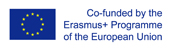This project has been funded with support from the European Commission.
This web site reflects the views only of the author, and the Commission cannot be held responsible for any use which may be made of the information contained therein.

Select language   >   IT EN RO PL LT

### Pythagoras theorem

Subject taught
- Math

Type of Product
- Lesson Plans

Language Skills Developed
- Interaction
- Listening
- Speaking
- Writing

Transferable/Scientific Skills Developed
Student will develop self-motivation, analytical skills, problem-solving skills, regularity in repeated reasoning.

Description
General aims
Student will be able to explain how Pythagoras theorem works, students will apply the Pythagorean Theorem using it to find the missing length of a triangle.

Student will be able to solve basic mathematical problems connected with Pythagoras theorem. He will know basic vocabulary in English connected with geometry. Student will develop positive learning habits such as organisational and problem-solving skills.

Linguistic aims:
- to read English with ease
- to understanding content
- to learn from written texts
- to master tools for gathering information
- to express understanding and experiences
- to enable students to understand topic correctly
- to translate words connected with Pythagoras theorem in English into the mother tongue using one’s own words.

Subject specific aims:

- student can explain how Pythagoras theorem works
- student is able to use Pythagoras theorem in math problems which are connected directly with a right triangle
- student knows how to name the longest side of right triangle
- student identifies and applies the Pythagorean Theorem to find the missing hypotenuse of a triangle
- student identifies and applies the Pythagorean Theorem to find the missing leg of a triangle
- student gains confidence in their math skills
- student has unlimited access to free educational content one can master at their own pace
- student looks for and regularity in repeated reasoning
- student is better prepared for standardized testing.

Suggested Technology: Computer for Presenter, Basic Calculators, LCD Projector

Target group age:
Students from primary school (13-14 years old), before using the resource they need to be able to solve two-step equations, calculate and estimate square roots, evaluate expressions or equations with single digit exponents. Students also have to be familiarized with basic geometry. What is more, this lesson plan can be used by anyone who wants to know more about Pythagoras theorem, also by grade teachers who wants to teach math bilingually.

Level of competence in English (CEFR)
B1/B1+

Time required to use the resource with the students:
The teacher can use the resource with the students for one lessons. Lesson lasts 1 hour 30 minutes.

How to use it
Before using the lesson plan during one’s classes, the students should be able to do basic operation with powers, roots, equations and know basics of geometry.
The teacher asks students questions in English which should be displayed during the lesson. These questions shuld be discussed at the beginning, middle and end of the lesson.

“How many degrees has an angle which we define as a right angle?”
“What kind of triangle is a right triangle?”
“Which side of right angle is called hypotenuse? ”
“How can I tell which side is hypotenuse?” “How does the length of the hypotenuse compare to the length of a leg?”
“What is the Pythagorean Theorem in your own words?”
“What is the purpose of the Pythagorean Theorem?”
“How to solve hypotenuse?”
“How to figure out one side of the right triangle?”
“How to simplify square root?”
The students give the answers with the examples. The teacher should give them feedback and show students more examples on the black table. The teacher highlights some new words for students in English and students should find the translation these words into the mother tongue and write down the new words in English in the notebook with the right translation in their own words. The words which should be taken into consideration are: right triangle, triangle, squared, hypotenuse, Pythagoras, Pythagorean Theorem, missing length, missing side, apply Pythagorean Theorem, unknown side lengths, leg.
The teacher uses Pythagorean Theorem PowerPoint.ppt the to teach the lesson. The lesson plan has detailed instructions how to teach step by step.
Guided practice can be achieved through the use of the Pythagorean Theorem Worksheet.doc , which is one of the attachments.
Attachments:
Pythagorean_Theorem_Worksheet.docx